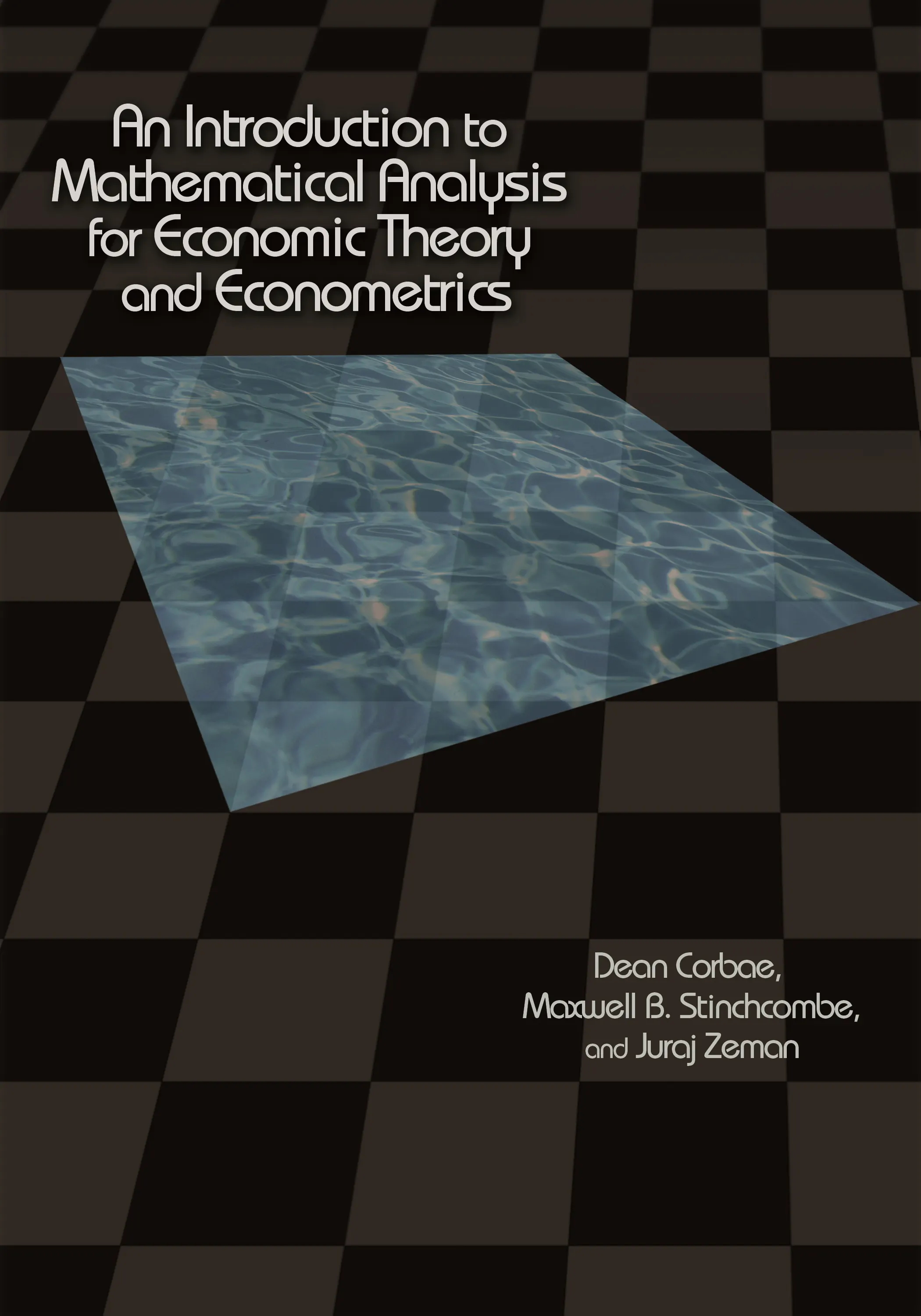#### An Introduction to Mathematical Analysis for Economic Theory and Econometrics

Dean Corbae

##### ebooks

Many of our ebooks are available for purchase from these online vendors:

Many of our ebooks are available through library electronic resources including these platforms:

# An Introduction to Mathematical Analysis for Economic Theory and Econometrics## Hardcover

Price:
\$115.00 / £90.00
ISBN:
Published:
Mar 9, 2009
2009
Pages:
688
Size:
7 x 10 in.
Illus:
55 line illus.

Providing an introduction to mathematical analysis as it applies to economic theory and econometrics, this book bridges the gap that has separated the teaching of basic mathematics for economics and the increasingly advanced mathematics demanded in economics research today. Dean Corbae, Maxwell B. Stinchcombe, and Juraj Zeman equip students with the knowledge of real and functional analysis and measure theory they need to read and do research in economic and econometric theory.

Unlike other mathematics textbooks for economics, An Introduction to Mathematical Analysis for Economic Theory and Econometrics takes a unified approach to understanding basic and advanced spaces through the application of the Metric Completion Theorem. This is the concept by which, for example, the real numbers complete the rational numbers and measure spaces complete fields of measurable sets. Another of the book’s unique features is its concentration on the mathematical foundations of econometrics. To illustrate difficult concepts, the authors use simple examples drawn from economic theory and econometrics.

Accessible and rigorous, the book is self-contained, providing proofs of theorems and assuming only an undergraduate background in calculus and linear algebra.

• Begins with mathematical analysis and economic examples accessible to advanced undergraduates in order to build intuition for more complex analysis used by graduate students and researchers

• Takes a unified approach to understanding basic and advanced spaces of numbers through application of the Metric Completion Theorem

• Focuses on examples from econometrics to explain topics in measure theory